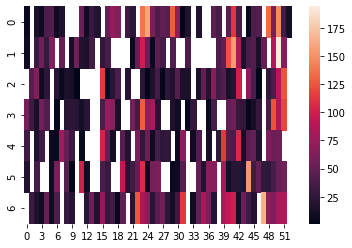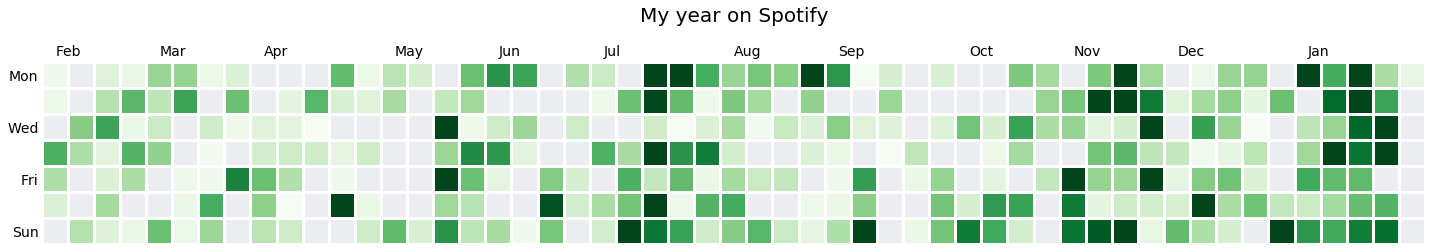← Back to blog

Inspired by Nerudista’s post in the Tacos de Datos website (in spanish) I asked Spotify for my data and started making some plots with them. If you want to do the same, head over to this page; once you request it, it will take a couple of days to be available. In the meantime, you can use this Google Colab, I’ve created a subset of my data for you to play with.

Among all the information you will receive, there will be some files named following this pattern: `StreamingHistoryXX.json`, and these are the ones we’ll use throughout this post.

## The data

The files mentioned above files contain something like this:

``````[
{
"endTime" : "2019-02-04 17:14",
"artistName" : "MGMT",
"trackName" : "Time to Pretend",
"msPlayed" : 261000
},
{
"endTime" : "2019-02-04 17:18",
"artistName" : "MGMT",
"..." ``````

Where the values are:

• `endTime`: Day and time in which a song finished, in UTC format.
• `artistName`: Name of the artist of the song.
• `trackName`: Name of the song.
• `msPlayed`: For how long (in milliseconds) a song was played.

To load these this data into a DataFrame we’ll need this tiny function:

``````from glob import glob
import json
import pandas as pd

history = []
for file in sorted(glob("StreamingHistory*.json")):
history = pd.DataFrame(history)
history["endTime"] = pd.to_datetime(history["endTime"])
return history

Which should give us something like this once we execute it:

endTimeartistNametrackNamemsPlayed
02019-02-04 17:14:00MGMTTime to Pretend261000
12019-02-04 17:18:00MGMTWhen You Die263880
22019-02-04 17:23:00Mr DoovesSuper Smash Bros. Brawl Main Theme (From “Super Smash Bros. Brawl”) [A Cappella]201518

## Histogram.

I’ve always been a fan of the way GitHub shows developer contributions, and the data we got from Spotify seems like the perfect candidate to create something similar; however, we need to perform some transformations first.

Since we are not interested in the time each song finished we’ll get rid of the temporal part of `endTime`:

``streaming_history["date"] = streaming_history["endTime"].dt.floor('d')``

Then we’ll get a count of songs per day using `groupby`:

``````by_date = streaming_history.groupby("date")[["trackName"]].count()
by_date = by_date.sort_index()``````

For out plot, we’ll need to know which day of the week each date refers to, we can use the properties `week` and `weekday`:

``````by_date["weekday"] = by_date.index.weekday
by_date["week"] = by_date.index.week``````

By the end, our dataframe should look like this:

trackNameweekdayweek
date
2019-02-04506
2019-02-05816
2019-02-076036

We have almost everything we need, the next step is to get a contiuous sequence of numbers for each week. In the dataframe above the 6th week of 2016 must be the week 0, the 7th must be the week 1… I can’t think of a better way to do it than a `for` loop:

``````week = 0
prev_week = by_date.iloc["week"]
continuous_week = np.zeros(len(by_date)).astype(int)
sunday_dates = []
for i, (_, row) in enumerate(by_date.iterrows()):
if row["week"] != prev_week:
week += 1
prev_week = row["week"]
continuous_week[i] = week
by_date["continuous_week"] = continuous_week
trackNameweekdayweekcontinuous_week
date
2019-02-045060
2019-02-058160
2019-02-0760360

Our next step is to generate a matrix with `days ✕ weeks` as dimensions, where each one of the entries will be the number of songs we listened to in that day and week:

``````songs = np.full((7, continuous_week.max()+1), np.nan)

for index, row in by_date.iterrows():
songs[row["weekday"]][row["continuous_week"]] = row["trackName"]``````

Now we could just plot the matrix `songs` using `seaborn`:

``````fig = plt.figure(figsize=(20,5))
ax = plt.subplot()
sns.heatmap(songs, ax = ax)``````

But the result is not that great:If we want it to look better we still need some code, the first thing to do is to clean the axis labels:

``````min_date = streaming_history["endTime"].min()
first_monday = min_date - timedelta(min_date.weekday())
mons = [first_monday + timedelta(weeks=wk) for wk in range(continuous_week.max())]
x_labels = [calendar.month_abbr[mons.month]]
x_labels.extend([
calendar.month_abbr[mons[i].month] if mons[i-1].month != mons[i].month else ""
for i in range(1, len(mons))])

y_labels = ["Mon", "", "Wed", "", "Fri", "", "Sun"]``````

The X axis labels are far more complicated than the Y ones; this is because unlike Y, the X axis is not fixed nor continuous, as such, they need to calculated based on the data. If you want a more detailed explanation, tell me in the comments or at me on twitter at @feregri_no).

After doing this we’ll perform some modifications with colours and the axis:

``````fig = plt.figure(figsize=(20,5))
ax = plt.subplot()

ax.xaxis.tick_top()
ax.tick_params(axis='both', which='both',length=0)
ax.set_facecolor("#ebedf0")
fig.patch.set_facecolor('white')``````

And finally, we can use seaborn’s `heatmap` again, this time with a few extr arguments that I will explain later:

``````sns.heatmap(songs, linewidths=2, linecolor='white', square=True,
vmin=0, vmax=100, cbar=False, ax=ax)

ax.set_yticklabels(y_labels, rotation=0)
ax.set_xticklabels(x_labels, ha="left")``````

The arguments are as follows:

• `songs`: our matrix with shape `days ✕ weeks` with the counts of songs per day,
• `linewidths`: the size of the spacing between each patch,
• `linecolor`: the colour of the spacing between each patch,
• `square`: this tells the function that we want to keep the aspect ratio `1:1` for each patch,
• `mask`: a very interesting argument, it will help us “mask” the patches for which there is no recorded value, this argument should be a boolean matrix of the same dimensions as the data being plotted, where each `True` means that that specific value must be masked,
• `cmap`: the colormap to be used, luckily for us, the value “Greens” matches with the colour palette chosen by GitHub,
• `vmin`: the value that should be considered as the minimum among our values,
• `vmax`: the value that should be considered as the maximum among our values, I’d consider 100 to be the maximum, even though my record sits as 190 in a day!
• `cbar`: a boolean value to indicate whether we want to show the colour bar that usually comes with the heatmap,
• `ax`: the axes our plot should be plotted on.

And voilà, our plot is ready:It is up to you to modify the plot, may be by adding information about the number of songs, or show the colorbar… a great idea would be to recreate this plot in a framework such as D3.js, but that may well belong to another post. Again, feel free to head over to this Colab and contact me via twitter @feregri_no.